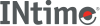﻿ Bessel functionsINtime SDK Help
Bessel functions

Computes the Bessel function.

```#include <math.h>

double j0 (double x);

double j1 (double x);

double jn (int n, double x);

double y0 (double x);

double y1 (double x);

double yn (int n, double x);
```

#### Parameters

`x`
Value must be positive for `y0`, `y1`, and `yn`.
`n`
Integer order.

#### Remarks

These functions are commonly used in the mathematics of electromagnetic wave theory.

#### Return Values

`j0`, `j1`, and `jn` return the result of Bessel functions of the first kind: orders 0, 1, and n, respectively.

`y0`, `y1`, and `yn` return the result of Bessel functions of the second kind: orders 0, 1, and `n`, respectively. If `x` is negative, functions set errno to EDOM, print a DOMAIN error message to stderr, and return -HUGE_VAL.

These functions does not return standard ANSI domain or range errors.

#### Requirements

Versions Defined in Include Link to
INtime 3.0 intime/rt/include/math.h math.h clib.lib